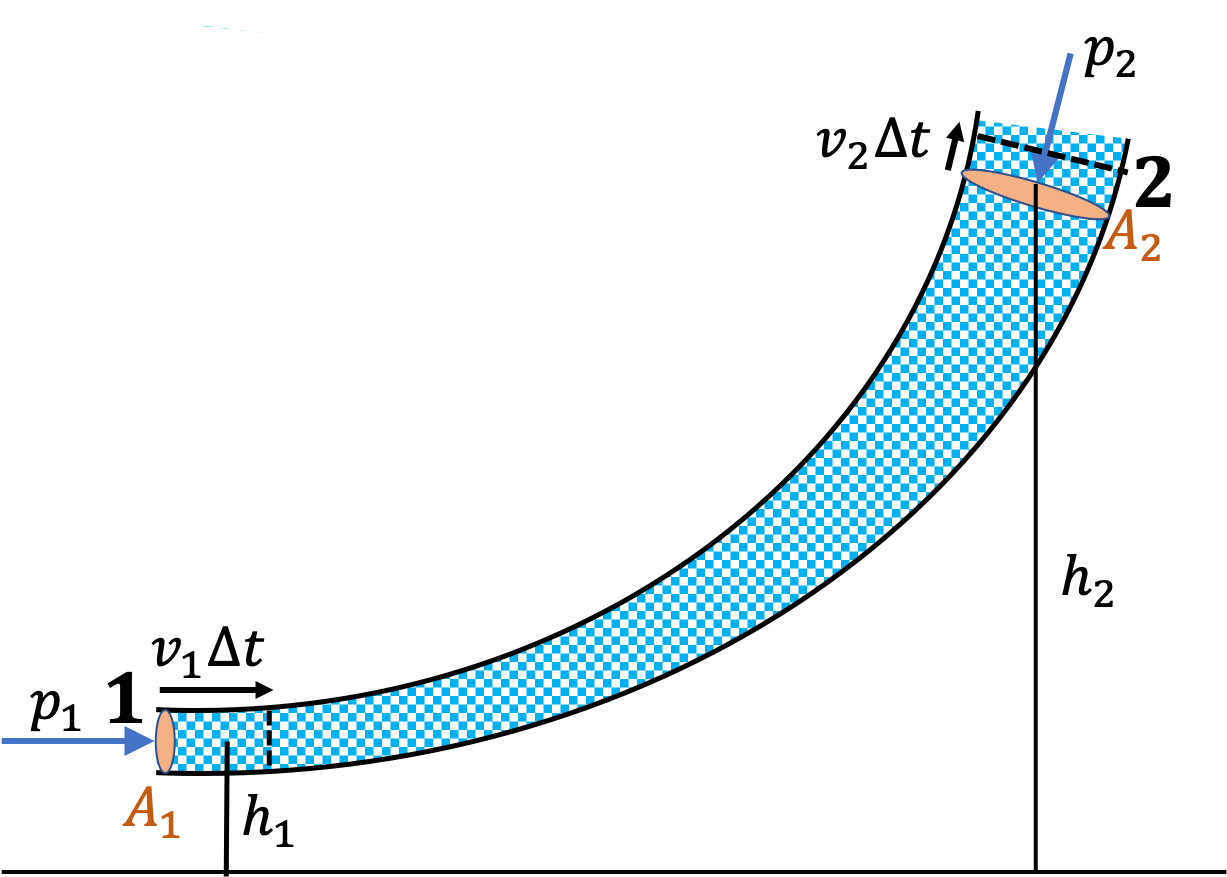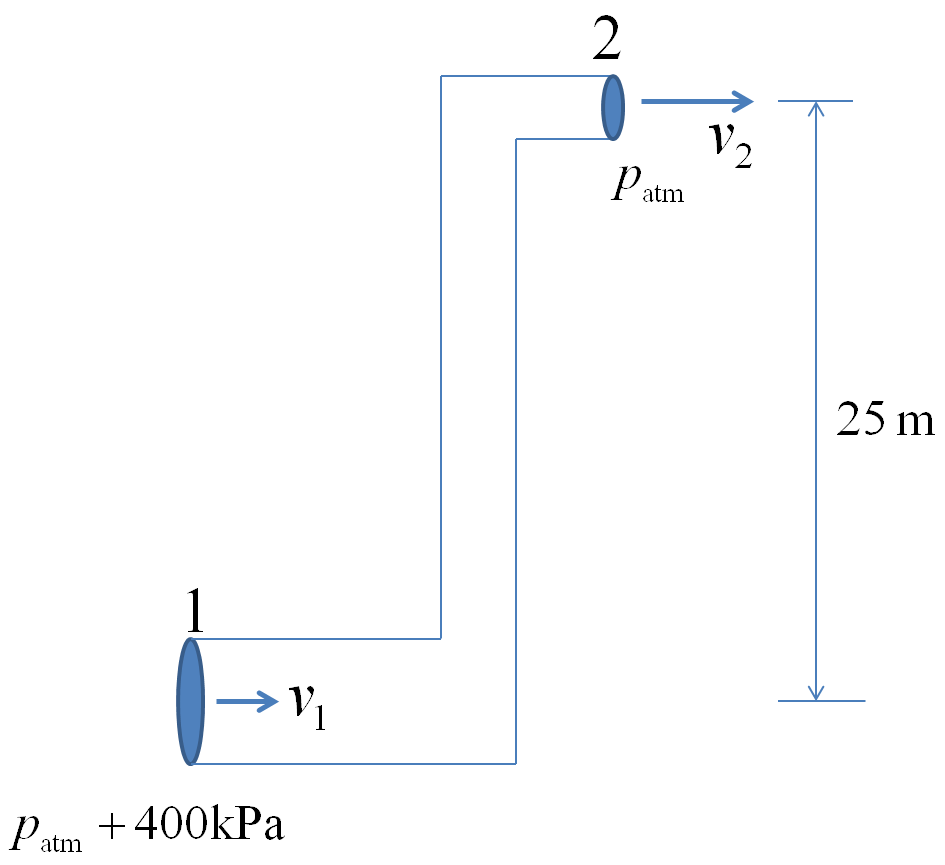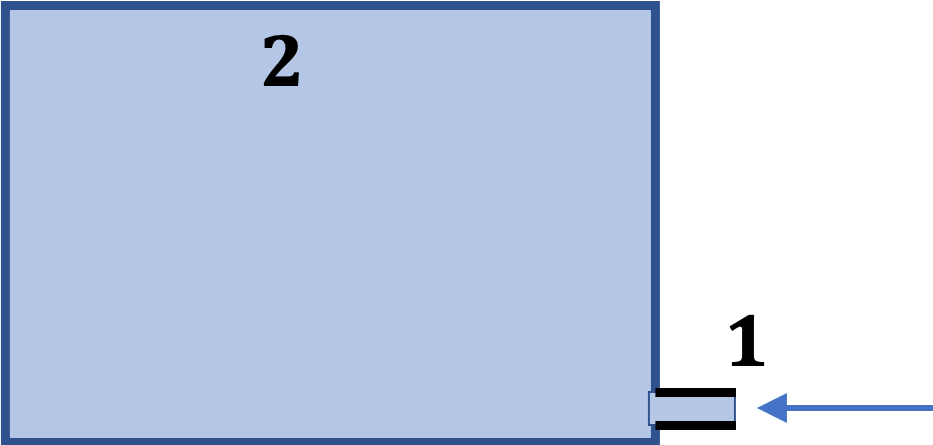## Section18.2Bernoulli's Equation

We will see below that if flow is viscous, then molecules drag each other down and fluid loses energy. However, if we can ignore viscosity, we expect energy to be conserved.

Applying conservation of energy to the fluid between two points along a flow Daniel Bernoulli (1700-1782) found a relation between flow speeds and pressures at the two ends. This relation is called Bernoulli's equation. It has many applications as we will see below.

Consider a fluid flowing through a pipe that may have different cross-sections and different heights along the way as shown in Figure 18.2.1.Figure 18.2.1. The fluids outside of the fluid between 1 and 2 are considered external to the system. During a time interval $\Delta t$ some energy comes into the system by work of pressure $p_1$ and material flow there. During the same interval, energy leaves the system at 2 due to work against $p_2$ and flow of material. The gain and loss of energy must equal in magnitude due to the conservation of energy in a non-viscous system.

Our system will be the fluid between points marked 1 and 2. The fluid before point 1 pushes onto the system doing positive work on the system, while the fluid after point 2 does negative work on the system. At point 1, it could even be the pump that is pushing the fluid in the pipe; in that case, work will be done by the pump.

The incoming fluid at 1 brings in kinetic and potential energies in the 1-to-2 space and the departing fluid at 2 reduces energy.

The work by $p_1$ at end 1 is

\begin{equation*} \vec F \cdot \Delta \vec r \longrightarrow (p_1 A_1)(v_1\Delta t). \end{equation*}

At 1, fluid outside of the system bring in kinetic energy

\begin{equation*} \dfrac{1}{2}mv^2 \longrightarrow \dfrac{1}{2} (\rho A v_1\Delta t) v_1^2, \end{equation*}

and potential energy,

\begin{equation*} mgh\longrightarrow (\rho A_1 v_1\Delta t) g h_1. \end{equation*}

We work out corresponding quantities at 2 similarly. Summarizing, processes at 1 increase the energy by

\begin{equation*} p_1 A_1 v_1\Delta t + \dfrac{1}{2}\rho A v_1\Delta t v_1^2 + \rho A_1 v_1\Delta t g h_1, \end{equation*}

and processes at 2 decrease the energy by

\begin{equation*} p_2 A_2 v_2\Delta t + \dfrac{1}{2}\rho A v_2\Delta t v_2^2 + \rho A_2 v_2\Delta t g h_2. \end{equation*}

Due to the incompressibility of fluid, we also have

\begin{equation*} A_1 v_1 = A_2 v_2. \end{equation*}

Equating the increase and decrease amounts, using the last equation, and canceling out the common factors give us the Bernoulli's equation.

\begin{equation} p_1 + \dfrac{1}{2}\rho v_1^2 + + \rho g h_1 = p_2 + \dfrac{1}{2}\rho v_2^2 + + \rho g h_2.\tag{18.2.1} \end{equation}

This says that along the flow we have a constant of motion.

\begin{equation} p + \dfrac{1}{2}\rho v^2 + + \rho g h = \text{constant}.\tag{18.2.2} \end{equation}

Water is to be pumped from the basement of a tall building such that $15\text{ l/min}$ of water must come out froma faucet of inner diameter $2.5\text{ cm}$ at a height $30\text{ m}\text{.}$ The water is being pumped into a pipe of diameter $5.0\text{ cm} \text{.}$ Assume no branching, and if branching, then all other branches are closed off. Assume nonviscous flow.

(a) What are the speeds of flow in the (i) basement and at (ii) faucet?

(b) What is the pressure at the pump?

Hint

(a) Convert units of $Q$ and use $Q = Av$ and $A_1v_1 = A_2 v_2 \text{.}$ (b) Use Bernoulli's equation.

(a) (i) $0.128\text{ m/s}\text{,}$ (ii) $0.51\text{ m/s}\text{,}$ (b) $2.95\times 10^{5}\text{ Pa} \text{.}$

Solution 1 (a)

(a) First, we find the speed of flow at the faucet by using the volume flow rate given. We need to convert that into the SI units.

\begin{equation*} Q = 15\text{ l/min} \times \dfrac{10^{-3} \text{ m}^3\text{/l}} {60\text{ s/min}} = 2.5\times 10^{-4}\text{ m}^3\text{/s}. \end{equation*}

Let subscript 1 refer to the pipe at the pump and 2 to the pipe at the faucet. Then, $A_2 v_2 = Q$ gives

\begin{equation*} v_2 = \dfrac{Q}{A_2} = \dfrac{2.5\times 10^{-4}}{ \pi\times 0.0125^2}= 0.51\text{ m/s}. \end{equation*}

From $A_1 v_1 = A_2 v_2\text{,}$ we get

\begin{equation*} v_1 = \dfrac{A_2}{A_1}\, v_2 = \left( \dfrac{2.5}{5} \right)^2\, 0.51 = 0.128\text{ m/s}. \end{equation*}
Solution 2 (b)

(b) Bernoulli's equation applies here since flow is nonviscous. The pressure against which water comes out of faucet is the atmospheric pressure. Using 1 for pump's locaiton and 2 for the faucet's location, we will have

\begin{align*} p_1 \amp = p_2 + \dfrac{1}{2}\rho (v_2^2 - v_1^2) + \rho g (h_2-h1) \\ \amp = 1.013\times 10^{5} + 1000\left[ \dfrac{1}{2} (0.51^2 - 0.128^2) + 9.81\times 30 \right] \\ \amp = 2.95\times 10^{5}\text{ Pa}. \end{align*}

Consider a tank of water with a tap at depth $h$ from the surface in a container as shown in Figure 18.2.4. As the tap is opened what is the speed with which water will come out? Assume steady flow. Also assume that the speed of flow of water at the surface in the tank to be very nearly zero compared to the speed at the tap.

Hint

At the exit, think what pressure the fluid is pushing against; note that it's not $p_0+\rho g h\text{.}$

$\sqrt{2gh}$

Solution

To apply Bernoulli's equation, we consider two points in the fluid. Point 1 is at the surface and point 2 is just outside the valve at the spigot as shown in Figure 18.2.5.

Point 1: Since the area of cross-section at the surface is much larger than the opening, the speed of flow at the surface is much smaller than the speed at the opening. Therefore, I will assume that the speed at a point at the top of the tank may be set to zero without incurring significant error. The pressure at the surface is the atmospheric pressure $p_0$ since it is freely exposed to the atmosphere.

Point 2: The pressure against which the water comes out at the opening is also the atmospheric pressure $p_0$ and not $p_0+\rho gh$ as would be the case if water was not flowing! This aspect is somewhat disturbing to people first learning to think in terms of dynamics rather than static situation, especially after memorizing formulas of static fluid in the last chapter. The reason for the pressure at the exit to be equal to the atmospheric pressure has to do with the fact that the only the force due to the atmospheric pressure is opposing the flow coming out of the nozzle.

One way to figure out the dynamic pressure at the exit is to pretend that you are the fluid at the exit, and ask, “what force will you be pushing against?” When you think in this language, you would not be confused about the dynamic pressure at any point.

We now put the properties at the two points 1 and 2 in the Bernoulli's equation.

\begin{equation*} p_0 + 0+ \rho g h = p_0 + \dfrac{1}{2}\rho v_2^2 + 0. \end{equation*}

Therefore, the speed of water at the spigot is

\begin{equation*} v_2 = \sqrt{2gh} \end{equation*}

This formula is also called the Torricelli's theorem . It is interesting to note that the speed of a freely falling particle dropped from a height $h$ is given by the same formula.

A water tank is drained by a pipe that varies in cross-section, with $5\text{ cm}$ diameter at the tank (point 2) and a diameter of $1\text{ cm}$ at the exit (point 1) as shown in Figure 18.2.7. Point 2 is $30\text{ cm}$ above point 1. The surface of water in the tank is $50\text{ cm}$ above point 1.

(a) Find the speed of flow at point 1.

(b) Find the volume rate of flow at 1.

(c) Find the volume rate of flow at point 2.

(d) Find pressure at point 2.

Hint

(a) Use Bernoulli's equation. (b) $Q=A_1v_1\text{,}$ (c) $A_1v_1 = A_2v_2\text{.}$

(a) $3.13 \ \text{m/s} \text{,}$ (b) $246\ \text{cm}^3/\text{s} \text{,}$ (c) $12.5\ \text{cm/s}\text{,}$ (d) $p_{\text{atm}} - 2450\ \text{Pa}\text{.}$

Solution 1 (a)

We take the point 1 as the reference for the height, set it height zero. Let us denote the pressure, speed and height at points marked 0, 1, and 2 by attaching subscripts to $p\text{,}$ $v\text{,}$ and $h$ respectively. Bernoulli's equation applies to any two points along the flow and in the following we will apply this equation between the two pairs here 0 to 1 and 1 to 2.

(a) The Bernoulli's equation for 0 $\rightarrow$ 1 gives

\begin{equation} p_0 + \dfrac{1}{2}\rho v_0^2 + \rho g h_0 = p_1 + \dfrac{1}{2}\rho v_1^2 + \rho g h_1. \label{eq-ch13-f-d-ex4-1}\tag{18.2.3} \end{equation}

Since the point 0 is open to the atmosphere, the pressure at that point is the atmospheric pressure. Similarly the pressure at point 1 is also atmospheric pressure since the fluid is exiting against the atmosphere.

\begin{align*} p_0 \amp = p_{\text{atm}}, \\ p_1 \amp = p_{\text{atm}}. \end{align*}

The tank is assumed to be wide compares to the pipes and the flow at point 0 can be assumed to be of low speed such that we can take $v_0$ to be zero.

\begin{equation*} v_0 \approx 0. \end{equation*}

The height $h_1$ is zero by the choice for heights and the height $h_0$ with respect to point 1 is $50\text{ cm}\text{.}$

\begin{align*} \amp h_0 = 50\ \text{cm },\\ \amp h_1 = 0. \end{align*}

Putting these in Eq. (18.2.3) gives us an equation that can be simplified to

\begin{align*} v_1 \amp = \sqrt{2 g h_0} = \sqrt{2\times 981\ \text{cm/s}^2 \times 50\text{ cm} }, \\ \amp = 313 \ \text{cm/s} = 3.13 \ \text{m/s}. \end{align*}
Solution 2 (b)

(b)

\begin{align*} \text{Volume rate of flow}\amp = A_1 v_1 = \dfrac{\pi}{4}\times (1\ \text{cm})^2 \times 313\ \text{cm/s}\\ \amp = 246\ \text{cm}^3/\text{s}. \end{align*}
Solution 3 (c)

(c) The conservation equation $A_1v_1 = A_2 v_2$ gives

\begin{align*} v_2 \amp = \left(\dfrac{d_1}{d_2}\right)^2 v_1, \\ \amp = \left(\dfrac{1}{5}\right)^2 \times 313\ \text{cm/s} = 12.5\ \text{cm/s}. \end{align*}
Solution 4 (d)

(d) To find $p_2$ we can apply Bernoulli's equation between 0 and 2 or 1 and 2. We will use 1 and 2 pair.

\begin{equation*} p_2 + \dfrac{1}{2}\rho v_2^2 + \rho g h_2 = p_1 + \dfrac{1}{2}\rho v_1^2 + \rho g h_1. \end{equation*}

In this equation we already know the following.

\begin{align*} \amp h_1 = 0 \\ \amp v_1 = 3.13 \ \text{m/s}\\ \amp p_1 = p_{\text{atm}} = 1.013 \times 10^{5}\ \text{Pa}\\ \amp h_2 = 0.3\ \text{m}\\ \amp v_2 = 0.125\ \text{m/s} \end{align*}

Putting these values we solve for $p_2\text{.}$

\begin{equation*} p_2 = p_{\text{atm}} - 2450\ \text{Pa}. \end{equation*}

Water is pumped from the ground floor of a high-rise building to a 10th floor apartment $25\text{ meters}$ above. A gauge pressure at the pump reads $400\text{ kPa}$ and the pipe on the 10th floor is open to atmosphere. The pipe on the ground floor is $5\text{ cm}$ in diameter and that in the apartment is only $2\text{ cm}$ in diameter. Find speeds of flow at (a) the ground floor and (b) the tenth floor.Hint

Set up Bernoulli equation with point 1 at the ground floor and point 2 at the tenth floor.

(a) $2.85\text{ m/s}\text{,}$ (b) $17.8\text{ m/s}\text{.}$

Solution

(a) and (b). It is almost always helpful to sketch out the physical situation of the problem as shown in the figure.

We will be using the volume conservation equation and Bernoulli's equation to relate the properties at point 1 and 2.

\begin{align} \amp A_1 v_1 = A_2 v_2. \label{eq-ch13-f-d-ex-5-1}\tag{18.2.4}\\ \amp p_2 + \dfrac{1}{2}\rho v_2^2 + \rho g h_2 = p_1 + \dfrac{1}{2}\rho v_1^2 + \rho g h_1. \label{eq-ch13-f-d-ex-5-2}\tag{18.2.5} \end{align}

We have the following quantities known. I will assign symbols to work with before putting in the numbers at the end.

\begin{align*} \amp h_2-h_1 = 20\ \text{m} \equiv h.\\ \amp p_1 - p_2 = 400\ \text{kPa}\equiv \Delta p\\ \amp \dfrac{A_1}{A_2} = \left(\dfrac{d_1}{d_2}\right)^2 = 2.5^2 = 6.25 \equiv \alpha. \end{align*}

We need only the ratio of the areas of cross-section. Putting $v2 = \alpha v_1$ from Eq. (18.2.4) in Eq. (18.2.5) and dividing out by $\rho$ leads to

\begin{equation*} \dfrac{\Delta p}{\rho} + \dfrac{1}{2} v_1^2 = \dfrac{1}{2} \alpha^2 v_1^2 + g h. \end{equation*}

(a) This equation can be rearranged and solved for $v_1\text{.}$

\begin{align*} v_1 \amp = \sqrt{\dfrac{2}{\alpha^2 - 1} \left[ \dfrac{\Delta p}{\rho} - g h \right]}, \\ \amp = 2.85\ \text{m/s}. \end{align*}

(b) Putting back in Eq. (18.2.4) will give $v_2 = \alpha^2 v_1 = 17.8$ m/s.

A large water tank is filled with water to the top, and sealed off at the top so that it is not exposed to the atmospheric pressure. A small orifice is opened at the bottom. Determine the minimum water height in the tank for the water to leak at all.Hint

When you seal off the top, the pressure there would not be atmospheric pressure.

$10.34\text{ m}.$

Solution

When you seal off the top, the pressure there would not be atmospheric pressure. The water in the tank is supported by the push by the atmosphere at the opened orifice at the bottom until the height in the tank is greater than the critical height. At the critical height, the pressure at the top of the enclosed tank is zero.

Let us denote a point in the opened orifice as point 1 and the top point of water in the tank as point 2. We apply Bernoulli's equation.

\begin{equation*} p_2 + \frac{1}{2}\rho v_2^2 + \rho g h_2 = p_1 + \frac{1}{2}\rho v_1^2 + \rho g h_1. \end{equation*}At the critical height $h_c$ we will have the following values.

\begin{align*} \amp h_1 = 0,\\ \amp v_1 = 0\ \ \textrm{(just when starts to flow)}\\ \amp p_1 = p_{\textrm{atm}} = 1.013 \times 10^{5}\ \textrm{Pa}\\ \amp h_2 = h_c\ \ \textrm{(the critical height)}\\ \amp v_2 = 0. \end{align*}

This gives

\begin{equation*} \rho g h_c = p_{\textrm{atm}}. \end{equation*}

Therefore

\begin{equation*} h_c = \frac{p_{\textrm{atm}}}{\rho g} = 10.34\ \textrm{m}. \end{equation*}